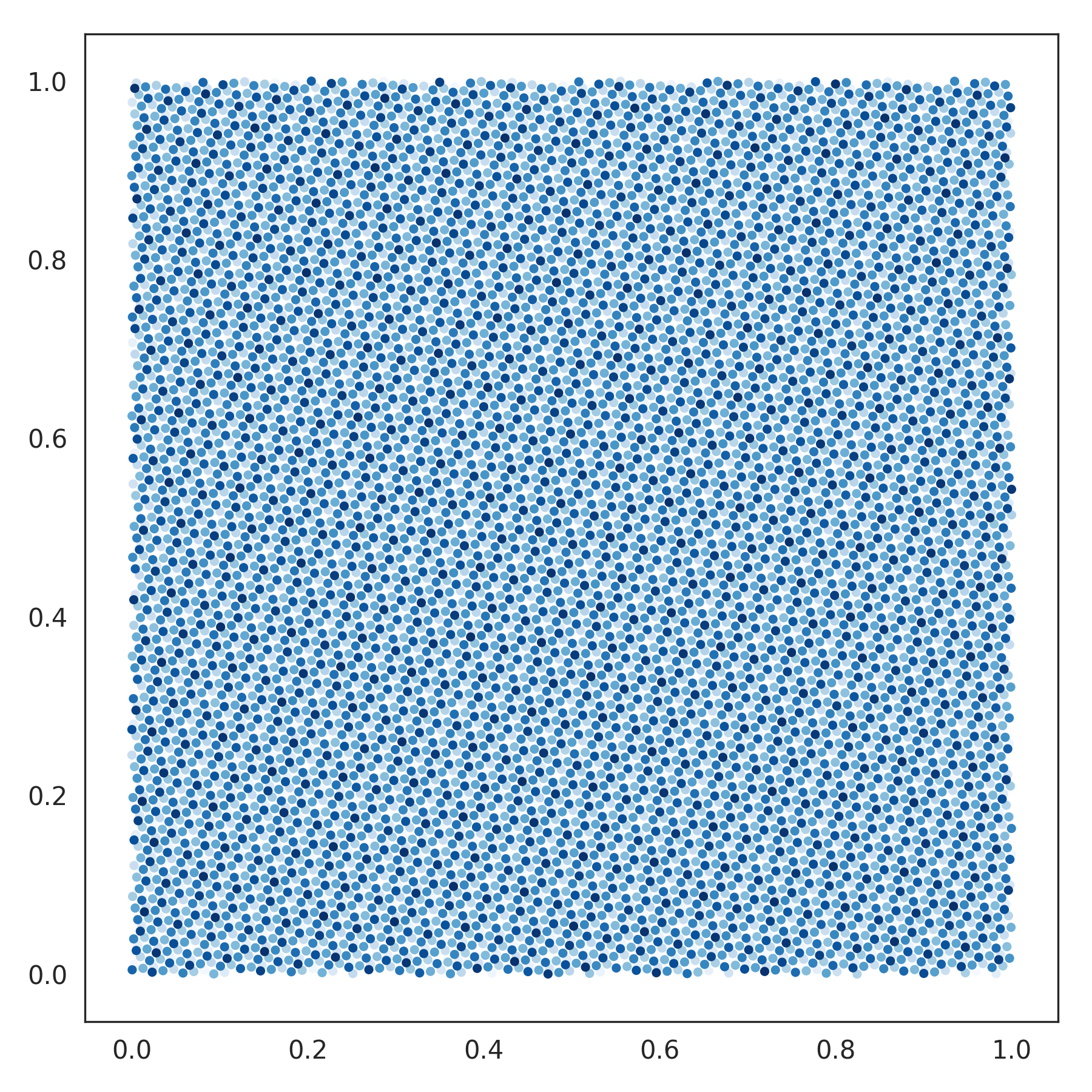nengolib.stats.Rd¶

class nengolib.stats.Rd[source]

Rd sequence for quasi Monte Carlo sampling the [0, 1]–cube.

This is similar to np.random.uniform(0, 1, size=(num, d)), but with the additional property that each d–dimensional point is uniformly scattered.

This is based on the tutorial and code from . For d=2 this is often called the Padovan sequence. 

References

Examples

>>> from nengolib.stats import Rd
>>> rd = Rd().sample(10000, 2)
>>> import matplotlib.pyplot as plt
>>> plt.figure(figsize=(6, 6))
>>> plt.scatter(*rd.T, c=np.arange(len(rd)), cmap='Blues', s=7)
>>> plt.show()Methods

 sample(n[, d, rng]) Samples n points in d dimensions.